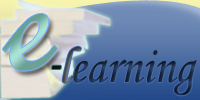Home Technical Mathematics Languages Science Social Science Art Literature Arabic Islamic Studies C.R.K HistoryMusicVisual Art Clothing/Textile Home Management Shorthand
 Further Mathematics Paper 2, Nov/Dec. 2013
 Questions: 1 2 3 4 5 6 7 8 9 10 11 12 13 14 15 16 17 18 Main
General Comments
Weakness/Remedies
Strength
Question 10

Question 10

Function f and g are defined over the set R of real numbers by f : x  32 + 2 and
g : x  , x  2.
Find:

1. the range of f when -3 ≤ x ≤ 4;
2. g-1, the inverse of g;
3. g-1 of (-2).
_____________________________________________________________________________________________________
Observation

This question was also reported to be attempted by majority of the candidates and their performance was described as fair.  Candidates were reported to have performed better in part (a) than in any other part of the question.

In part (a), since the function was quadratic, the minimum/maximum value would either be at the turning points or at the end points of the domain i.e. -3 ≤ x ≤ 4.  Therefore candidates were expected to substitute the end points of the domain -3≤ x ≤ 4 to obtain f(-3) = 29, f(4) = 50.  The minimum value of the function was obtained by differentiating the function to obtain f1(x) = 6   At the turning
point  = 0. f(0) = 2.  Therefore, 2 is the minimum value of the function and since x = 0 is part of the given interval, the range of f = 2≤ f() ≤ 50.

In part (b), candidates were expected to show that if g-1 is the inverse of g, then g(g-1(x)) =  i.e.  = .  Solving this equation gave g-1 = ,  x  1.  g-1 of (-2) =   =   = .

 Powered by Sidmach Technologies(Nigeria) Limited . Copyright © 2015 The West African Examinations Council. All rights reserved.